• 词语相似度计算词义相似度计算在很多领域中都有广泛的应用，例如信息检索、信息抽取、文本分类、词义排歧、基于...同义词词林扩展版《同义词词林》是梅家驹等人于1983年编纂而成，这本词典中不仅包括了一个词语的...
词语相似度计算词义相似度计算在很多领域中都有广泛的应用，例如信息检索、信息抽取、文本分类、词义排歧、基于实例的机器翻译等等。国内目前主要是使用知网和同义词词林来进行词语的相似度计算。本文主要是根据《基于同义词词林的词语相似度计算方法—田久乐》论文中所提出的分层算法实现相似度计算，程序采用Java语言编写。同义词词林扩展版《同义词词林》是梅家驹等人于1983年编纂而成，这本词典中不仅包括了一个词语的同义词，也包含了一定数量的同类词，即广义的相关词。《同义词词林扩展版》是由哈尔滨工业大学信息检索实验室所重新修订所得。该版收录词语近7万条，全部按意义进行编排，是一部同义类词典。同义词词林按照树状的层次结构把所有收录的词条组织到一起，把词汇分成大、中、小三类，大类有12个，中类有97个，小类有1400个。每个小类里都有很多的词，这些词又根据词义的远近和相关性分成了若干个词群(段落)。每个段落中的词语又进一步分成了若干个行，同一行的词语要么词义相同，要么词义有很强的相关性。《同义词词林》提供了5层编码，第1级用大写英文字母表示；第2级用小写英文字母表示；第3级用二位十进制整数表示；第4级用大写英文字母表示；第5级用二位十进制整数表示。例如：Cb30A01= 这里 这边 此地 此间 此处 此Cb30A02# 该地 该镇 该乡 该站 该区 该市 该村Cb30A03@ 这方分层及编码表如下所示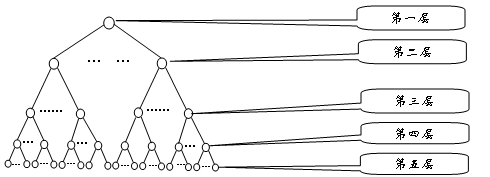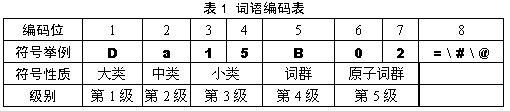由于第5级有的行是同义词，有的行是相关词，有的行只有一个词，分类结果需要特别说明，可以分出具体的3种情况。使用特殊符号对这3种情况进行区别对待，所以第8位的标记有3种，分别是“=”代表“相等”、“同义”；“#”代表“不等”、“同类”，属于相关词语；“@”代表“自我封闭”、“独立”，它在词典中既没有同义词，也没有相关词。在对文本内容进行相似度计算中，采用该论文中给出的计算公式，两个义项的相似度用Sim表示若两个义项不在同一棵树上，Sim(A,B)=f若两个义项在同一棵树上：若在第2层分支，系数为a Sim(A,B)=1*a*cos*(n*π/180)((n-k+1)/n)若在第3层分支，系数为b Sim(A,B)=1*1*b*cos*(n*π/180)((n-k+1)/n)若在第4层分支，系数为c Sim(A,B)=1*1*1*c×cos*(n*π/180)((n-k+1)/n)若在第5层分支，系数为d Sim(A,B)=1*1*1*1*d*cos*(n*π/180)((n-k+1)/n)当编码相同，而只有末尾是“#”时，那么认为其相似度为e。例如Ad02B04# 非洲人 亚洲人 则其相似度为e。其中n是分支层的节点分支总数，k是两个分支间的距离。如：人 Aa01A01= 和 少儿 Ab04B01=以A开头的子分支只有14个，分别是Aa*——An*，而不是以A开头的所有结点的个数；在第2层，人的编码是a，少儿的编码是b所以k=1。该文献中给出的参数值为a=0.65，b=0.8，c=0.9，d=0.96，e=0.5，f=0.1。如：人 Aa01A01= 和 少儿 Ab04B01=由于A开头的分支个数为14个，所以n=14；在第2层，人的编码是a，少儿的编码是b所以k=1。Java代码实现package edu.shu.similarity.cilin;import com.google.common.base.Preconditions;import lombok.extern.log4j.Log4j2;import org.apache.commons.io.IOUtils;import org.apache.commons.lang3.StringUtils;import org.junit.Test;import java.io.IOException;import java.io.InputStream;import java.util.*;import static java.lang.Math.PI;import static java.lang.Math.cos;/*** * Created with IntelliJ IDEA. 2015/8/2 21:54* * * ClassName:WordSimilarity* * * Description:* "=" 代表 相等 同义 * "#" 代表 不等 同类 属于相关词语 * "@" 代表 自我封闭 独立 它在词典中既没有同义词, 也没有相关词 * ** @author Wang Xu* @version V1.0.0* @since V1.0.0*/@Log4j2//注意使用Log4j2的注解，那么在pom中必须引入2.x版本的log4j，如果使用Log4j注解，pom中引入1.x版本的log4j//相应的配置文件也要一致，2.x版本配置文件为log4j2.xml，1.x版本配置文件为log4j.xmlpublic class WordSimilarity{/*** when we use Lombok's Annotation, such as @Log4j** @Log4j * public class LogExample {* }* * will generate:* public class LogExample {* private static final org.apache.logging.log4j.Logger log = org.apache.logging.log4j.Logger.getLogger(LogExample.class);* }* *///定义一些常数先private final double a = 0.65;private final double b = 0.8;private final double c = 0.9;private final double d = 0.96;private final double e = 0.5;private final double f = 0.1;private final double degrees = 180;//存放的是以词为key，以该词的编码为values的List集合，其中一个词可能会有多个编码private static Map> wordsEncode = new HashMap>();//存放的是以编码为key，以该编码多对应的词为values的List集合，其中一个编码可能会有多个词private static Map> encodeWords = new HashMap>();/*** 读取同义词词林并将其注入wordsEncode和encodeWords*/private static void readCiLin(){InputStream input = WordSimilarity.class.getClassLoader().getResourceAsStream("cilin.txt");List contents = null;try {contents = IOUtils.readLines(input);} catch (IOException e) {e.printStackTrace();log.error(e.getMessage());}for (String content : contents) {content = Preconditions.checkNotNull(content);String[] strsArr = content.split(" ");String[] strs = Preconditions.checkNotNull(strsArr);String encode = null;int length = strs.length;if (length > 1) {encode = strs;//获取编码}ArrayList encodeWords_values = new ArrayList();for (int i = 1; i < length; i++) {encodeWords_values.add(strs[i]);}encodeWords.put(encode, encodeWords_values);//以编码为key，其后所有值为valuefor (int i = 1; i < length; i++) {String key = strs[i];if (wordsEncode.containsKey(strs[i])) {ArrayList values = wordsEncode.get(key);values.add(encode);//重新放置回去wordsEncode.put(key, values);//以某个value为key，其可能的所有编码为value} else {ArrayList temp = new ArrayList();temp.add(encode);wordsEncode.put(key, temp);}}}}/*** 对外暴露的接口，返回两个词的相似度的计算结果** @param word1* @param word2* @return 相似度值*/public double getSimilarity(String word1, String word2){//如果比较词没有出现在同义词词林中，则相似度为0if (!wordsEncode.containsKey(word1) || !wordsEncode.containsKey(word2)) {return 0;}//获取第一个词的编码ArrayList encode1 = getEncode(word1);//获取第二个词的编码ArrayList encode2 = getEncode(word2);double maxValue = 0;//最终的计算结果值，取所有相似度里面结果最大的那个for (String e1 : encode1) {for (String e2 : encode2) {log.info(e1);log.info(e2);String commonStr = getCommonStr(e1, e2);int length = StringUtils.length(commonStr);double k = getK(e1, e2);double n = getN(commonStr);log.info("k--" + k);log.info("n--" + n);log.info("length--" + length);double res = 0;//如果有一个以“@”那么表示自我封闭，肯定不在一棵树上，直接返回fif (e1.endsWith("@") || e2.endsWith("@") || 0 == length) {if (f > maxValue) {maxValue = f;}continue;}if (1 == length) {//说明在第二层上计算res = a * cos(n * PI / degrees) * ((n - k + 1) / n);} else if (2 == length) {//说明在第三层上计算res = b * cos(n * PI / degrees) * ((n - k + 1) / n);} else if (4 == length) {//说明在第四层上计算res = c * cos(n * PI / degrees) * ((n - k + 1) / n);} else if (5 == length) {//说明在第五层上计算res = d * cos(n * PI / degrees) * ((n - k + 1) / n);} else {//注意不存在前面七个字符相同，而结尾不同的情况，所以这个分支一定是8个字符都相同，那么只需比较结尾即可if (e1.endsWith("=") && e2.endsWith("=")) {//说明两个完全相同res = 1;} else if (e1.endsWith("#") && e2.endsWith("#")) {//只有结尾不同，说明结尾是“#”res = e;}}log.info("res :" + res);if (res > maxValue) {maxValue = res;}}}return maxValue;}/*** 判断一个词在同义词词林中是否是自我封闭的，是否是独立的** @param source* @return*/private boolean isIndependent(String source){Iterator iter = wordsEncode.keySet().iterator();while (iter.hasNext()) {String key = iter.next();if (StringUtils.equalsIgnoreCase(key, source)) {ArrayList values = wordsEncode.get(key);for (String value : values) {if (value.endsWith("@")) {return true;}}}}return false;}/*** 根据word的内容，返回其对应的编码** @param word* @return*/public ArrayList getEncode(String word){return wordsEncode.get(word);}/*** 计算N的值，N表示所在分支层分支数，如：人 Aa01A01= 和 少儿 Ab04B01=，以A开头的子分支只有14个* 这一点在论文中说的非常不清晰，所以以国人的文章进行编码真是痛苦** @param encodeHead 输入两个字符串的公共开头* @return 经过计算之后得到N的值*/public int getN(String encodeHead){int length = StringUtils.length(encodeHead);switch (length) {case 1:return getCount(encodeHead, 2);case 2:return getCount(encodeHead, 4);case 4:return getCount(encodeHead, 5);case 5:return getCount(encodeHead, 7);default:return 0;}}public int getCount(String encodeHead, int end){Set res = new HashSet();Iterator iter = encodeWords.keySet().iterator();while (iter.hasNext()) {String curr = iter.next();if (curr.startsWith(encodeHead)) {String temp = curr.substring(0, end);if (res.contains(temp)) {continue;} else {res.add(temp);}}}return res.size();}/*** @param encode1 第一个编码* @param encode2 第二个编码* @return 这两个编码对应的分支间的距离，用k表示*/public int getK(String encode1, String encode2){String temp1 = encode1.substring(0, 1);String temp2 = encode2.substring(0, 1);if (StringUtils.equalsIgnoreCase(temp1, temp2)) {temp1 = encode1.substring(1, 2);temp2 = encode2.substring(1, 2);} else {return Math.abs(temp1.charAt(0) - temp2.charAt(0));}if (StringUtils.equalsIgnoreCase(temp1, temp2)) {temp1 = encode1.substring(2, 4);temp2 = encode2.substring(2, 4);} else {return Math.abs(temp1.charAt(0) - temp2.charAt(0));}if (StringUtils.equalsIgnoreCase(temp1, temp2)) {temp1 = encode1.substring(4, 5);temp2 = encode2.substring(4, 5);} else {return Math.abs(Integer.valueOf(temp1) - Integer.valueOf(temp2));}if (StringUtils.equalsIgnoreCase(temp1, temp2)) {temp1 = encode1.substring(5, 7);temp2 = encode2.substring(5, 7);} else {return Math.abs(temp1.charAt(0) - temp2.charAt(0));}return Math.abs(Integer.valueOf(temp1) - Integer.valueOf(temp2));}/*** 获取编码的公共部分字符串** @param encode1* @param encode2* @return*/public String getCommonStr(String encode1, String encode2){int length = StringUtils.length(encode1);StringBuilder sb = new StringBuilder();for (int i = 0; i < length; i++) {if (encode1.charAt(i) == encode2.charAt(i)) {sb.append(encode1.charAt(i));} else {break;}}int sbLen = StringUtils.length(sb);//注意第三层和第五层均有两个字符，所以长度不可能出现3和6的情况if (sbLen == 3 || sbLen == 6) {sb.deleteCharAt(sbLen - 1);}return String.valueOf(sb);}@Testpublic void testGetN(){readCiLin();int a = getN("A");System.out.println(a);}@Testpublic void testGetK(){int k = getK("Aa01A01=", "Aa01A01=");System.out.println(k);}@Testpublic void testGetCommonStr(){String commonStr = getCommonStr("Aa01A01=", "Aa01A03=");System.out.println(commonStr);}@Testpublic void testGetSimilarity(){readCiLin();double similarity = getSimilarity("人民", "国民");System.out.println("人民--" + "国民:" + similarity);similarity = getSimilarity("人民", "群众");System.out.println("人民--" + "群众:" + similarity);similarity = getSimilarity("人民", "党群");System.out.println("人民--" + "党群:" + similarity);similarity = getSimilarity("人民", "良民");System.out.println("人民--" + "良民:" + similarity);similarity = getSimilarity("人民", "同志");System.out.println("人民--" + "同志:" + similarity);similarity = getSimilarity("人民", "成年人");System.out.println("人民--" + "成年人:" + similarity);similarity = getSimilarity("人民", "市民");System.out.println("人民--" + "市民:" + similarity);similarity = getSimilarity("人民", "亲属");System.out.println("人民--" + "亲属:" + similarity);similarity = getSimilarity("人民", "志愿者");System.out.println("人民--" + "志愿者:" + similarity);similarity = getSimilarity("人民", "先锋");System.out.println("人民--" + "先锋:" + similarity);}@Testpublic void testGetSimilarity2(){readCiLin();double similarity = getSimilarity("非洲人", "亚洲人");System.out.println(similarity);double similarity1 = getSimilarity("骄傲", "仔细");System.out.println(similarity1);}}说明，词语相似度是个数值，一般取值范围在[0，1]之间，在原论文中，使用cos函数计算主要是将值归一化到[0，1]之间，可以将cos函数看作是一个调节因子。testGetSimilarity的测试结果如下所示：人民--国民:1.0人民--群众:0.9576614882494312人民--党群:0.8978076452338418人民--良民:0.7182461161870735人民--同志:0.6630145969121822人民--成年人:0.6306922220793977人民--市民:0.5405933332109123人民--亲属:0.36039555547394153人民--志愿者:0.22524722217121346人民--先锋:0.18019777773697077本文使用的是同义词词林的扩展版，而原论文使用的是同义词词林，由于两者存在微小差距，所以本文计算结果与论文中的计算结果存在稍许误差，如果算法没错，这是可以理解的！以上仅为个人理解，如若发现错误，欢迎大家积极留言指正！目前整个项目已经推送到GitHub上了，地址点我。注意在文章末尾所注的参考资料中的链接里面的计算方法在求n的时候存在错误，代码我不确定是否是其自己实现，望莫要受其误导！
展开全文• 同义词词林 可用于机器翻译 消除句子歧义 信息检索 数据时txt的 整理的很规则 很好用啊 同义词词林 可用于机器翻译 消除句子歧义 信息检索 数据时txt的 整理的很规则 很好用啊
• 学习NLP的同学在进行同义词替换、关键词扩展等操作时常用到同义词词林，哈工大社会计算与信息检索研究中心同义词词林扩展版，较为适用，有需要的可下载使用
• 哈工大同义词词林-最新版2020版 值得大家下载，推荐大家 不错 NLP 词林 同义词nlp
• 我想免费的，CSDN没有免费项，这回坑爹了。这是哈工大的同义词词林，一起学习
• 由于《同义词词林》著作时间较为久远，且之后没有更新，所以原书中的某些词语成为生僻词，而很多新词又没有加入。有鉴于此，哈尔滨工业大学信息检索实验室利用众多词语相关资源，并投入大量的人力和物力，完成了一部...
• 同义词词林》是梅家驹等人于1983年编纂而成，年代较为久远，对于目前的使用不太适合，哈工大实验室基于该词林进行扩展，完成了词林扩展版。 下载地址：https://www.ltp-cloud.com/download/ 二、使用说明 扩展...
一、前言

《同义词词林》是梅家驹等人于1983年编纂而成，年代较为久远，对于目前的使用不太适合，哈工大实验室基于该词林进行扩展，完成了词林扩展版。
下载地址：https://www.ltp-cloud.com/download/

二、使用说明

扩展版同义词词林分为5层结构，

随着级别的递增，词义刻画越来越细，到了第五层，每个分类里词语数量已经不大，很多只有一个词语，已经不可再分，可以称为原子词群、原子类或原子节点。不同级别的分类结果可以为自然语言处理提供不同的服务，例如第四层的分类和第五层的分类在信息检索、文本分类、自动问答等研究领域得到应用。有研究证明，对词义进行有效扩展，或者对关键词做同义词替换可以明显改善信息检索、文本分类和自动问答系统的性能。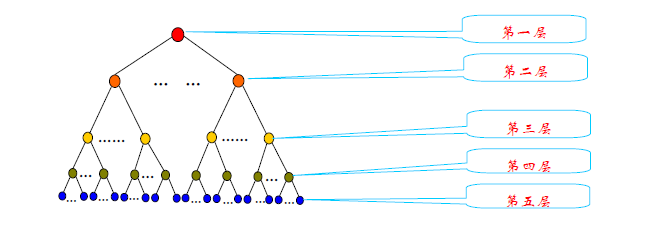下载后的词典文件如下所示：

Aa01A01= 人 士 人物 人士 人氏 人选
Aa01A02= 人类 生人 全人类
Aa01A03= 人手 人员 人口 人丁 口 食指
Aa01A04= 劳力 劳动力 工作者
Aa01A05= 匹夫 个人


上述文件编码代表含义见图：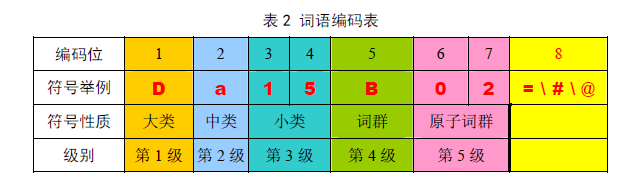表中的编码位是按照从左到右的顺序排列。第八位的标记有3 种，分别是“=”、“#”、“@”， “=”代表“相等”、“同义”。末尾的“#”代表“不等”、“同类”，属于相关词语。末尾的“@”代表“自我封闭”、“独立”，它在词典中既没有同义词，也没有相关词。

三、其他

1、上文引用均来自哈工大扩展词林说明文档
2、一个获取同义词的python库：https://github.com/huyingxi/Synonyms
展开全文• 哈工大的同义词词林扩展版，用于获取近义词的python代码和Java代码
• 哈工大词林：老婆 妻子翻译：老婆->wife->妻子缩写：北京大学 北大利用输入词，生成所有的候选短语, 然后利用翻译进行校验，比如北大和北京大学的翻译都是Peking University.百科infobox：可以发现北京大学的...
本文介绍同义词构造的若干方法。Enjoy！哈工大词林：老婆 妻子翻译：老婆->wife->妻子缩写：北京大学 北大利用输入词，生成所有的候选短语, 然后利用翻译进行校验，比如北大和北京大学的翻译都是Peking University.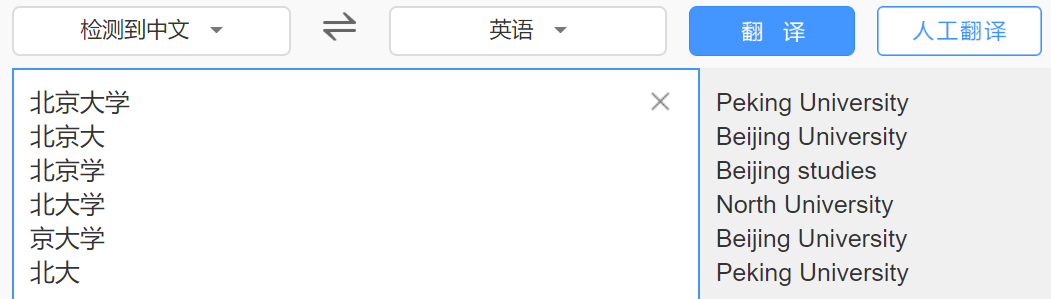百科infobox：可以发现北京大学的infobox有简称这个属性，那么可以认为属性值北大和北京大学是同义词。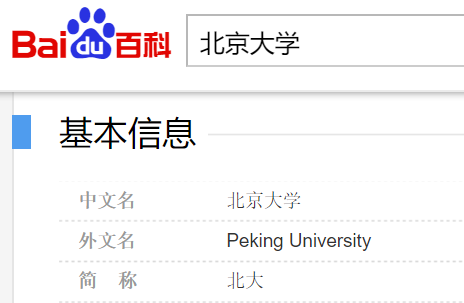常见的同义词属性有 ['别称', '别名', '又名', '又称', '也叫', '也称', '昵称', '俗名', '名称', '标准名称', '本名', '学名', '全称', '简称', '缩写', '缩略词', '简写', '英文名', '英文名称']维基第一段维基第一段黑体的词汇可以认为是标题的同义词。另外关于近义词可以参考https://github.com/huyingxi/Synonyms​github.com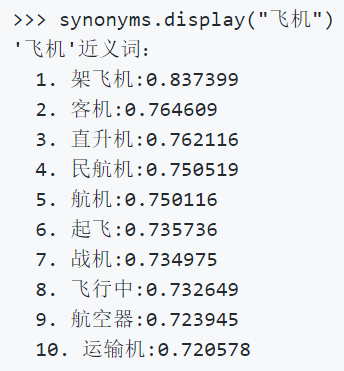Happy Reading, Happy Learning!
展开全文• 本例是基于《哈工大信息检索研究中心同义词词林扩展版》做的，其中详细分析了将源文件差拆分成五层结构，并对同义词计算，进行了跟踪分析。 其中根目录下的Synonymy是项目文件|||Synonymy/text-file中的origin.txt...
• 同义词词林哈工大扩展版 在做搜索和数据挖掘和自然语言处理时有用，也可以用来计算词语的相似度
• 我主要根据《中文信息学报》2016年9⽉第30卷第5期中的《基于路径与深度的同义词林词语相似度计算》提及到的计算公式进行了代码实现，并根据其最终的结果对原设计进行了微调。import osimport csvimport reclass Node...
我主要根据《中文信息学报》2016年9⽉第30卷第5期中的《基于路径与深度的同义词林词语相似度计算》提及到的计算公式进行了代码实现，并根据其最终的结果对原设计进行了微调。import osimport csvimport reclass Node(object):def __init__(self,id,children,parent,words):self.id = id.copy()self.children = []self.parent = Noneself.words = words.copy()self.level = len(id) if len(id) < 5 else 5def append_node(self,child):self.children.append(child)child.parent = selfreturndef normalize(cilin):cilin_to_list = cilin.split()id_list = re.findall(r"\D|\d\d",cilin_to_list.strip())word_list = cilin_to_list.copy()del word_listnew_node = Node(id_list,None,None,word_list)return new_nodedef findparent(Root,sub_node,depth):if len(Root.children) == 0:return Root #if it have no kids,then it will have a new kidselse:for child in Root.children: #else check every kids, the true parent must be among themif(sub_node.id[depth]==child.id[depth]):return findparent(child,sub_node,depth+1)return Rootdef add2dict(word_dict,node):for word in node.words:if word in word_dict:word_dict[word].append(node)else:word_dict[word] = []word_dict[word].append(node)returndef AnalyzeCilin(filename):cilin_node_list = []word_dict = {}with open(filename,"r",encoding="gbk") as f:cilinlist = f.readlines()for cilin in cilinlist:cilin_node_list.append(normalize(cilin))Root = Node(['Root'],None,None,['Root'])for cilin_node in cilin_node_list:temp = findparent(Root,cilin_node,0)if(None == temp):print("error")print(cilin_node.id)return None,Nonetemp.append_node(cilin_node)if(cilin_node.level == 5):add2dict(word_dict,cilin_node)return Root,word_dictdef GenerateParents(word_node,Root):parents_list = []sub_node = word_nodewhile not sub_node == Root:parents_list.append(sub_node)sub_node = sub_node.parentparents_list.append(Root)return parents_listdef find_sub_tree(word1,word2,Root,word_dict):maxdepth = 0tar_node = Rootsub_word1 = Rootsub_word2 = RootK = 0for word1_node in word_dict[word1]:for word2_node in word_dict[word2]:word1_parents = GenerateParents(word1_node,Root)word2_parents = GenerateParents(word2_node,Root)for i in range(6):if(word1_parents[i] == word2_parents[i]):if(maxdepth < word1_parents[i].level):maxdepth = word1_parents[i].leveltar_node = word1_parents[i]sub_word1 = word1_nodesub_word2 = word2_nodereturn tar_node,sub_word1,sub_word2def CalculateK(sub_root,word1_node,word2_node):for i in range(word1_node.level if word1_node.level < word2_node.level else word2_node.level):if not (word1_node.id[i] == word2_node.id[i]):if (word1_node.id[i].isalpha()):return (abs(ord(word1_node.id[i]) - ord(word2_node.id[i])))elif(word1_node.id[i].isdigit()):return (abs(int(word1_node.id[i]) - int(word2_node.id[i])))else:print("Error")return 0return 0def Sim(word1,word2,Root,word_dict):weight = [8,6,4,1.5,0.5]sub_root,word1_node,word2_node = find_sub_tree(word1,word2,Root,word_dict)K = CalculateK(sub_root,word1_node,word2_node)if(sub_root == word1_node and sub_root == word2_node):if(sub_root.id[-1] == '#'):return 0.98elif(sub_root.id[-1] == '='):return 1Comm = 0.9for i in range(sub_root.level):Comm = Comm + weight[i]Diff = (float(weight[sub_root.level]) * K) / len(sub_root.children)for i in range(sub_root.level,word1_node.level):Diff = Diff + weight[i]for i in range(sub_root.level,word2_node.level):Diff = Diff + weight[i]print(word1,word2,"Comm=",Comm,"Diff",Diff)return Comm / (Comm + Diff)def SimCalculate(Root,filename,word_dict):test_word = []with open(filename,'r',encoding="gbk") as csvfile:csv_reader = csv.reader(csvfile)for row in csv_reader:test_word.append(row)for test in test_word:if(test in word_dict and test in word_dict):Similarity = Sim(test,test,Root,word_dict)test.append(Similarity)else:test.append("不在库中")print(test,test,test[-1])return test_worddef Save2File(SimResult,filename):headers = ['Word1','Word2','Similarity']with open(filename,'w',encoding="gbk") as csvfile:csv_writer = csv.writer(csvfile)csv_writer.writerow(headers)csv_writer.writerows(SimResult)returnif __name__ == '__main__':Root,word_dict = AnalyzeCilin("cilin_扩展版.txt")SimResult = SimCalculate(Root,"测试用例.csv",word_dict)Save2File(SimResult,"测试用例的相似度(最终版).csv")未完待续，代码的进一步说明待到考完期末考试再补。
展开全文• 哈工大的同义词词林，应该是上个世纪的产物，里面的词比较老旧，但好歹也能用同义词词林的作用，跟word2vec的获取相近词函数比较类似，这两者发挥的功效比较，看具体的应用吧1. 首先下载包含同义词的txt，CSDN上有...
• 由于《同义词词林》著作时间较为久远，且之后没有更新，所以原书中的某些词语成为生僻词，而很多新词又没有加入。有鉴于此，哈尔滨工业大学信息检索实验室利用众多词语相关资源，并投入大量的人力和物力，完成了一部...
• self complement of Sentence Similarity compute based on cilin, hownet, simhash, wordvector,vsm models，基于同义词词林，知网，指纹，字词向量，向量空间模型的句子相似度计算。
• 基于同义词词林的词语相似度计算方法 介绍了根据同义词词林来计算词语相似度的方法。在做实验室感觉比用本体要方便一点
• 用java实现的哈工大同义词词林相似度计算，包含工程和文档，可以用来计算两个词之间的相似度，导入Myeclipse可以直接运行
• 哈尔滨工业大学信息检索实验室利用众多词语相关资源，并投入大量的人力和物力，完成了一部具有汉语大词表的《哈工大信息检索研究室同义词词林扩展版》。扩展版剔除了原版中的14,706个罕用词和非常用词，最终的词表...
• 同义词词林扩展版 # 收录词语近 7万条, 全部按意义进 行编排, 是一部同义类词典。哈工大信息检索研究室参照多部电子词典资源, 并按照人民日报语料库中 词语的出现频度, 只保留频度不低于 3的 (小规模语料的统计...
• 基于哈工大同义词词林的词语间相似度计算 局限：单纯使用同义词词林来计算相似度，如果词典中没有该词，就算不出相似度。 代码（在python3.6上正常运行） #!/usr/bin/env python3 # -*- coding: utf-8 -*- #2018...
• 共9994行 大类用大写英文字母表示; 中类用小写英文字母表示; 小类用二位十进制整数表示; 例： Ag02B02= 跛子 瘸子 拐子 跛脚 瘸腿 A为大类，g为中类，02为小类
• 哈工大社会计算与信息检索研究中心同义词词林扩展版，用于中文同义词归并非常有效，一般在NLP中作预处理降维。
• 完整的同义词词集，可用于分词，一份不可多得的同义词词典。
• 词语相似度分析（《知网》、同义词词林、word2vec）本篇对刚刚接触词语相似度分析的小白提供一个方向词义相似度计算方法分两种，一种是基于世界知识或某种分类体系的方法，例如基于《知网》、同义词词林的。...词语相似度分析 自然语言处理 word2vec
• 同义词词林扩展版与知网（Hownet）的词语相似度计算方法，词汇覆盖更多、结果更准确。